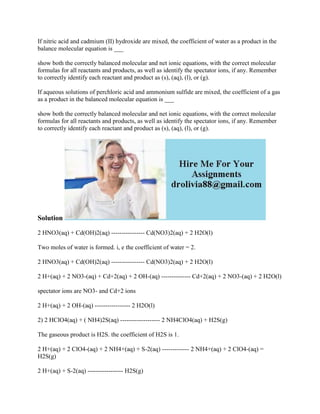Ce diaporama a bien été signalé.
Le téléchargement de votre SlideShare est en cours. ×

# If nitric acid and cadmium (II) hydroxide are mixed- the coefficient o.docx

Publicité
Publicité
Publicité
Publicité
Publicité
Publicité
Publicité
Publicité
Publicité
Publicité
PublicitéChargement dans…3
×

## Consultez-les par la suite

1 sur 2 Publicité

# If nitric acid and cadmium (II) hydroxide are mixed- the coefficient o.docx

If nitric acid and cadmium (II) hydroxide are mixed, the coefficient of water as a product in the balance molecular equation is ___
show both the correctly balanced molecular and net ionic equations, with the correct molecular formulas for all reactants and products, as well as identify the spectator ions, if any. Remember to correctly identify each reactant and product as (s), (aq), (l), or (g).
If aqueous solutions of perchloric acid and ammonium sulfide are mixed, the coefficient of a gas as a product in the balanced molecular equation is ___
show both the correctly balanced molecular and net ionic equations, with the correct molecular formulas for all reactants and products, as well as identify the spectator ions, if any. Remember to correctly identify each reactant and product as (s), (aq), (l), or (g).
Solution
2 HNO3(aq) + Cd(OH)2(aq) ---------------- Cd(NO3)2(aq) + 2 H2O(l)
Two moles of water is formed. i, e the coefficient of water = 2.
2 HNO3(aq) + Cd(OH)2(aq) ---------------- Cd(NO3)2(aq) + 2 H2O(l)
2 H+(aq) + 2 NO3-(aq) + Cd+2(aq) + 2 OH-(aq) -------------- Cd+2(aq) + 2 NO3-(aq) + 2 H2O(l)
spectator ions are NO3- and Cd+2 ions
2 H+(aq) + 2 OH-(aq) ----------------- 2 H2O(l)
2) 2 HClO4(aq) + ( NH4)2S(aq) ------------------- 2 NH4ClO4(aq) + H2S(g)
The gaseous product is H2S. the coefficient of H2S is 1.
2 H+(aq) + 2 ClO4-(aq) + 2 NH4+(aq) + S-2(aq) ------------- 2 NH4+(aq) + 2 ClO4-(aq) = H2S(g)
2 H+(aq) + S-2(aq) ----------------- H2S(g)
.

If nitric acid and cadmium (II) hydroxide are mixed, the coefficient of water as a product in the balance molecular equation is ___
show both the correctly balanced molecular and net ionic equations, with the correct molecular formulas for all reactants and products, as well as identify the spectator ions, if any. Remember to correctly identify each reactant and product as (s), (aq), (l), or (g).
If aqueous solutions of perchloric acid and ammonium sulfide are mixed, the coefficient of a gas as a product in the balanced molecular equation is ___
show both the correctly balanced molecular and net ionic equations, with the correct molecular formulas for all reactants and products, as well as identify the spectator ions, if any. Remember to correctly identify each reactant and product as (s), (aq), (l), or (g).
Solution
2 HNO3(aq) + Cd(OH)2(aq) ---------------- Cd(NO3)2(aq) + 2 H2O(l)
Two moles of water is formed. i, e the coefficient of water = 2.
2 HNO3(aq) + Cd(OH)2(aq) ---------------- Cd(NO3)2(aq) + 2 H2O(l)
2 H+(aq) + 2 NO3-(aq) + Cd+2(aq) + 2 OH-(aq) -------------- Cd+2(aq) + 2 NO3-(aq) + 2 H2O(l)
spectator ions are NO3- and Cd+2 ions
2 H+(aq) + 2 OH-(aq) ----------------- 2 H2O(l)
2) 2 HClO4(aq) + ( NH4)2S(aq) ------------------- 2 NH4ClO4(aq) + H2S(g)
The gaseous product is H2S. the coefficient of H2S is 1.
2 H+(aq) + 2 ClO4-(aq) + 2 NH4+(aq) + S-2(aq) ------------- 2 NH4+(aq) + 2 ClO4-(aq) = H2S(g)
2 H+(aq) + S-2(aq) ----------------- H2S(g)
.

Publicité
Publicité

### If nitric acid and cadmium (II) hydroxide are mixed- the coefficient o.docx

1. 1. If nitric acid and cadmium (II) hydroxide are mixed, the coefficient of water as a product in the balance molecular equation is ___ show both the correctly balanced molecular and net ionic equations, with the correct molecular formulas for all reactants and products, as well as identify the spectator ions, if any. Remember to correctly identify each reactant and product as (s), (aq), (l), or (g). If aqueous solutions of perchloric acid and ammonium sulfide are mixed, the coefficient of a gas as a product in the balanced molecular equation is ___ show both the correctly balanced molecular and net ionic equations, with the correct molecular formulas for all reactants and products, as well as identify the spectator ions, if any. Remember to correctly identify each reactant and product as (s), (aq), (l), or (g). Solution 2 HNO3(aq) + Cd(OH)2(aq) ---------------- Cd(NO3)2(aq) + 2 H2O(l) Two moles of water is formed. i, e the coefficient of water = 2. 2 HNO3(aq) + Cd(OH)2(aq) ---------------- Cd(NO3)2(aq) + 2 H2O(l) 2 H+(aq) + 2 NO3-(aq) + Cd+2(aq) + 2 OH-(aq) -------------- Cd+2(aq) + 2 NO3-(aq) + 2 H2O(l) spectator ions are NO3- and Cd+2 ions 2 H+(aq) + 2 OH-(aq) ----------------- 2 H2O(l) 2) 2 HClO4(aq) + ( NH4)2S(aq) ------------------- 2 NH4ClO4(aq) + H2S(g) The gaseous product is H2S. the coefficient of H2S is 1. 2 H+(aq) + 2 ClO4-(aq) + 2 NH4+(aq) + S-2(aq) ------------- 2 NH4+(aq) + 2 ClO4-(aq) = H2S(g) 2 H+(aq) + S-2(aq) ----------------- H2S(g)